## conference papersJOURNAL OFAPPLIEDCRYSTALLOGRAPHY
ISSN: 1600-5767
Volume 40| Part s1| April 2007| Pages s62-s67

## Field-dependent relaxation behavior of Co-ferrofluid investigated with stroboscopic time-resolved small-angle neutron scattering

aHahn-Meitner-Institut Berlin, Dept SF3, Glienicker Str. 100, D-14109 Berlin, Germany
*Correspondence e-mail: keiderling@hmi.de

(Received 22 August 2006; accepted 9 November 2006; online 19 December 2006)

In an external magnetic field, concentrated Co-ferrofluids form an inter-particle order revealing coexistence of a pseudo-crystalline hexagonal ordering of core-shell nanoparticles and segments of dipolar chains. The decay of this order after switching off the magnetic field is characterized by time constants of a few seconds. This relaxation behaviour has been investigated using a newly developed stroboscopic small-angle neutron scattering technique based on listmode data acquisition. This technique utilizes the fact that the process of creation and decay of the inter-particle order is reversible, thus allowing very good scattering statistics to be gained by stroboscopically repeating the process and superimposing the data from the individual cycles. This paper presents time constants determined for the decay of inter-particle order states created by different external magnetic fields. The decay is generally characterized by an exponential course, assigned to Brownian rotation of locally ordered domains, although in the very beginning it appears to take place faster, indicating a different decay mechanism. The time constants at fields > 0.1 T significantly increase, indicating a larger size of the ordered domains resulting from enhanced dipolar interactions.

### 1. Introduction

In previous polarized small-angle neutron scattering (SANSPOL) experiments on concentrated Co-ferrofluids, pronounced peaks in sixfold symmetry were observed in an external magnetic field B which disappear when the field is switched off. The peaks observed at the scattering vector Q1 were assigned to a pseudo-crystalline ordering where Co core-shell particles are arranged in hexagonal planes. The magnetic moments are aligned in the planes along the external magnetic field which corresponds to the  direction. However, the hexagonal c axis, defined by a peak at Q2, was found to be randomly distributed in a plane perpendicular to the magnetic field. The correlation length of the locally ordered domains was determined from the widths of the peaks to be of the order of 90 nm (Wiedenmann et al., 2003). In zero magnetic field, the observed Q−1 behaviour of the isotropic SANS intensities indicated that particle moments are spontaneously arranged in the attractive head-to-tail conformation in segments of uncorrelated chains formed by about 3 or 4 particles. The uncorrelated chain segments were found to be aligned along the direction of the magnetic field even at very low magnitudes of B. However, with increasing B the chains do not grow further in length. Instead lamellar arrangements are formed which become more and more correlated, giving rise to the broadened peaks (Wiedenmann, 2005; Wiedenmann & Heinemann, 2005).

As soon as the magnetic field is switched off, these locally ordered structures decay within a few seconds (Wiedenmann et al., 2006b). Due to the requirement of sufficient counting statistics, initially it had been impossible to investigate this transient state of the ferrofluid in a standard SANSPOL experiment. In a recent paper (Keiderling et al., 2006) we introduced a new time-resolved SANS technique, based on listmode data acquisition, recording full discrete (x, y, time) information for each single detected neutron. The usage of these data provides fully flexible control over the spatial and time resolution of the results after the experiments. For the present work, this time-resolved data acquisition technique has been combined with SANSPOL, allowing the investigation of the short-lived decay process by periodically repeating the switching off of the magnetic field and stroboscopically superimposing the scattering data from the individual cycles for very good statistics. The paper investigates the correlation between the strength of the external magnetic field and the time constant of the structural decay.

### 2. Experimental

A concentrated Co-ferrofluid MFT' with a Co content of 6 vol.% dispersed in L9 oil (Wiedenmann & Heinemann, 2005), contained in a Hellma quartz cell of 1 mm thickness, was placed in the homogeneous horizontal magnetic field of a standard electromagnet, applied perpendicular to the incoming beam. All measurements were performed with a fixed sample-to-detector distance of 4 m. Polarized neutron in-situ SANS measurements were performed at the V4 SANS instrument of HMI Berlin with various magnetic fields between 0.005 and 1 T. For each field, the scattering intensities were measured with the incident neutron beam polarization alternately parallel I(−) and antiparallel I(+) to the magnetic field. For each of these polarizations, listmode data were acquired with the field cycling between the two values field on' (0.005–1 T, depending on the particular experiment) and field off' (remanence < 0.005 T), as shown by the solid line in Fig. 1. For all measurements, a field on' time of 5 s and a field off' time of 15 s were used. Due to the limitations of the maximum slope of the magnet power supply (50 A s−1), the field on' and field off' phases were linked by an up-ramp and a down-ramp of the magnetic field (up to 3 s per ramp depending on the magnet current), creating a total cycle time of more than 20 s. For each measurement, approximately 200 to 1200 of these cycles were recorded, with a trigger marker placed at the end of the down-ramp intricately merged with the listmode data stream.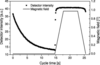Figure 1 Typical course of external magnetic field and total detector intensity I(−) − I(+) during the cycles (example: field on' ≃ 1 T).

After the experiments, the new listmode option (Keiderling et al., 2006) of the BerSANS software package (Keiderling, 2002), which is the standard software for raw data treatment, data reduction and visualization at the V4 SANS instrument of HMI Berlin, was applied to superimpose the raw data from the individual cycles. Using the trigger markers as reference points, the software regrouped the continuous stream of single detected neutrons into a set of two-dimensional histograms (time frames) with a spatial resolution of 128 × 128 cells each, which corresponds to the native resolution of the detector. For the width of each time frame a value of 100 ms was chosen, yielding a good compromise between the two contradictory requirements of a high number of frames for good time resolution and a low number of frames for good statistics within the single frames. After regrouping, the time frames were corrected for sample transmission and background scattering, and normalized to water scattering, also using the BerSANS software.

The method of stroboscopic superposition is only applicable for a reversible process. Therefore, the reversibility of the order creation and decay was verified for the ferrofluid in two different ways. First, for one selected time during field on' (at t = 20 s in Fig. 1) the corresponding time frame was recreated from the listmode data using only the first few cycles, and then again using only the last few cycles. Both these time frames were in good agreement with each other and with the time frame created for the same selected time from all cycles, indicating that the ordered state established in the sample during the short field on' phases remained the same even after many ordering/decay processes in the sample. Second, the same time frame created from all cycles was checked to be equal to the result of a standard SANSPOL measurement performed with a static external field of the same field strength, indicating that the kind of ordering achieved was the same in both cases.

### 3. Results and discussion

#### 3.1. General findings

For the investigated ferrofluid, the difference between the corrected and normalized SANSPOL intensities measured with neutron beam polarization parallel and antiparallel to the magnetic field,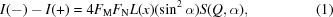represents the nuclear–magnetic interference term resulting solely from magnetic particles. L(x) = cosh(x) − 1/x is the Langevin function with x = Vcm0B/kT (Vc = core volume, m0 = saturation magnetization of Co). Q is the modulus of the scattering vector, Q = (4π/λ)sin(θ/2) where λ is the wavelength and θ is the scattering angle. α is the angle between the directions of the magnetic field B and the scattering vector Q. FM and FN are the magnetic and nuclear form factors, respectively, and S(Q, α) is the anisotropic structure factor (Wiedenmann, 2005). All intensities shown in the figures of this paper display this difference I(−) − I(+).

The dots in Fig. 1show the time correlation between the total intensity and the course of the external magnetic field. Each point represents the total intensity within one time frame, added up over all 128 × 128 cells of this frame. The on' phase with a duration of 5 s created the magnetic ordering in equilibrium. As the figure indicates, this ordering process took place extremely fast, in the case of higher fields reaching full order well before the external field reached its maximum. Hence the limiting factor for the measured ordering kinetics was given by the slope of the field-up ramp provided by the electromagnet (i.e. ≃ 0.18 s for 0 to 0.005 T, ≃ 3 s for 0 to 1 T), rather than by the native sample dynamics. We therefore investigated the ordering separately in an oscillating magnetic field and the results are presented in a different paper in this volume (Wiedenmann et al., 2006b).

A significant ordering was observed in the sample even at very low fields, and developed further with increasing field strength. To illustrate this, Fig. 2shows the intensities I(−) − I(+) during the field on' period measured at magnetic fields B = 0.005 T (a) and B = 1 T (b). A nearly complete removal of the ordering could only be achieved by running the magnet through a demagnetization loop with decreasing currents of alternating directions. For comparison, Fig. 2(c) gives the result of a separate static measurement performed after such demagnetization. However, no demagnetization could be performed during the stroboscopic recording of the cycles. Therefore, each new order creation at the beginning of a field on' period started from a residual structure that was retained by the remanence of the magnet (< 0.005 T) like Fig. 2(a), rather than from a complete disorder like Fig. 2(c).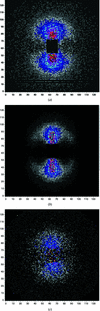Figure 2 Intensities I(−) − I(+) during field on' period at B = 0.005 T (a) and B = 1 T (b). For comparison: Intensity after nearly full demagnetization (c). The display colours have been normalized to the individual intensity ranges.

#### 3.2. Determination of decay time constants

Previous investigations of this ferrofluid sample have revealed the co-existence of a local pseudo-crystalline hexagonal order of core-shell nanoparticles and segments of dipolar chains aligned along the magnetic field. In the I(−) − I(+) plot, the hexagonal in-plane peaks at Q1 = 0.39 nm−1 are found at α = 30, 90, 150, 210, 270 and 330° while the inter-plane peaks are located at Q2 = 0.24 nm−1 at α = 90 and 270°, respectively. In the 90 and 270° directions the peaks at Q1 and Q2 merge and superimpose with the scattering contribution from dipolar chains, which follows a characteristic Q−1 dependence at low Q. The intensity of the latter contributions is much higher than the hexagonal peaks in the same directions, indicating that these segments of chains must be aligned along the magnetic field. Fig. 3(a) shows the same spectrum as Fig. 2(b), but highlights the above-mentioned directions.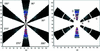Figure 3 Sectors used for radial averaging (a) and regions used from these sectors for calculation of total peak intensities (b).

In order to investigate the time-dependent behaviour of the peaks, in a first step radial averaging of the two-dimensional data was performed over the sectors displayed in Fig. 3(a), with a total sector width of 20°. The averaging was done for the 30° direction (including the opposite 210° direction for improved statistics) and for the 90° direction (including 270°). The results are shown in Fig. 4for the highest applied field of 1 T. For lower fields, the curves look similar and the same interpretations apply, with one exception mentioned below.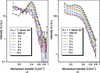Figure 4 Intensities I(−) − I(+), radially averaged over sector width 20° in the directions 30° (a) and 90° (b), during the field on' period with B = 1 T and at different times after switching off this field.

The finally complete disappearance of the interference peak in Fig. 4(a) (direction 30°) clearly reveals that the local hexagonal order is unstable and disintegrates during the first few seconds after switching off the field. During this disintegration, the peak position shifts slightly towards lower values of the scattering vector Q, indicating increasing distances of the particles within the planes during this decay. Interestingly, a new portion of intensity is created at very low Q after switching off the field which was not present during the field on' period. We assume that this intensity originates from a growing amount of dipolar chains. During field on', the chain segments existing at this time are fully aligned along the horizontal magnetic field, therefore producing a scattering intensity mainly in the 90° direction only. Shortly after switching off the field, these existing chains start to relax from their horizontal orientation into other directions. At the same time, the decay of the hexagonally ordered domains sets in, producing additional chain segments. Both processes contribute to the intensity rise observed. Beyond the intensity maximum, the chain segments gradually dissolve to smaller units, causing the observed intensity to decrease again. There is one significant difference in this behaviour that depends on the strength of the magnetic field. While the shift of the peak has always been observed, the delayed occurrence of an intensity maximum at very low Q after the trigger point was only observed at high fields. It was significant at B = 1 T (as shown in the figure), weaker at B = 0.333 T (not shown), but not observed at lower fields. From this it can be concluded that only in a high field can a significant amount of longer chain segments be conserved over a longer time period. More details on this effect will be given below, during the quantitative discussion of the decay process.

According to Fig. 4(b) (direction 90°), the inter-plane order reveals similar behaviour to the hexagonal one, with a decrease of intensity and a disappearing of the peak.

After this qualitative discussion, in the second step the time behaviour of the absolute peak intensities was investigated quantitatively. For this purpose, the sectors that yielded the radially averaged curves in Fig. 4were subdivided into regions of Q, A, B and C, as shown in Fig. 3(b), and the total intensity within the regions was calculated as a function of time. For direction 30°, region A covered the Q range below the peak, region B the main part of the peak itself, and region C the Q range above the peak. For direction 90°, region A covered the main part of the dipolar chain contribution at low Q, and regions B and C covered the long wing of the peak. Taking advantage of the symmetry in the two-dimensional scattering patterns, statistics were improved by including the respective regions at 150, 210 and 330° in the 30° direction, and the respective regions at 270° in the 90° direction.

For the longest part of the cycle, except in the very beginning immediately after the trigger, the time dependence of the intensity within each region was well fitted by a single exponential curve,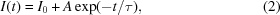where t is the time after the trigger point and I0 is the intensity for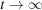. τ is interpreted as the characteristic relaxation time of the decay.

Fig. 5shows the time dependence of the intensities within the regions for B = 1 T. The curves clearly show the smooth and continuous decay of the peaks in both directions (30° region B, 90° region A).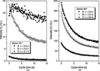Figure 5 Time-dependent intensities within regions A, B and C, and calculated decay time constants τ for directions 30° (a) and 90° (b) at B = 1 T.

In the 90° direction, the decay of the intensities in the wing of the peak (regions B and C) proceeds in a very similar way to the main peak region. This behaviour was found to be common for all investigated fields.

In the 30° direction, the most interesting region is A. Fig. 5(a) shows that at the highest field of B = 1 T the intensity still continues to rise for as much as 2 s after the trigger point. For this, one should bear in mind that the trigger point is already located at the very end of the down-ramp of the magnetic field (see Fig. 1), so that the total decay process has lasted longer than 2 s. Also, the time constant τ = 5.2 s for region A (fitted only to the decay part of this curve) turns out to be much longer than the time constant τ = 4.0 s obtained for the hexagonal peak in region B. This proves the stability of the fragments created after switching off the high field. At a lower field of B = 0.333 T (not shown), a smaller rise of the intensity in region A could be observed at the very beginning of the decay. At all other fields B < 0.333 T, the delayed maximum of the intensity curve in region A disappeared, and the curves looked similar to the one shown in Fig. 5(a) for region B. Obviously, these fields were too weak to produce large fragments that would last for a reasonable time. Finally, outside the hexagonal peak (region C) there was only very weak intensity for all fields, without any effects worth mentioning.

A comparison of the decay behaviour of the hexagonal in-plane peaks (30° region B) and the inter-plane contribution (90° region A) at B = 1 T shows that in spite of their very different intensities, both decay with the same time constant of τ = 4.0 s.

Fig. 6gives a total overview of the time constants τ fitted to the decay curves with equation (2). In the case of direction 30°, no reliable fit could be performed for regions A and C at small fields, due to the bad statistics caused by the low total intensities inside these regions. The inset of Fig. 6displays the typical course of the error bars, illustrated by direction 30° region B, which is representative for the other directions and regions as well. The relative error of the τ values calculated starts at ≃ 35% for the smallest field of 5 mT and reduces to ≃ 1% for the highest field of 1 T.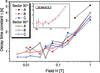Figure 6 Double-logarithmic plot of the decay time constants τ calculated for different magnetic fields. The inset figure displays the typical course of the error bars, illustrated by direction 30°, region B'.

The figure (in double-logarithmic plot) clearly shows that at smaller fields up to approximately 0.1 T there is a weak field dependence of the decay time constants. However at fields > 0.1 T, τ significantly increases, indicating a stabilization of the structures.

#### 3.3. Discussion of the down-ramp of the magnetic field

During the field down-ramp, the kinetics of disordering are hindered by the limited slope of the external magnetic field, as for the up-ramp discussed before. As can be seen in Fig. 1, at the end of the magnetic pulse the total intensity at the detector only starts to diminish when the field is already low. Therefore, it was not possible to include this time range into the fit of the decay time constants.

To get at least a basic quantitative idea of this very first part of the decay process prior to the defined trigger point, an extrapolation of the exponential decay curves towards times before the trigger point (i.e. to cycle time < 0 s in Fig. 1) has been performed. For these extrapolated curves it has then been determined at what times before the trigger point they would reach the level of the detector intensity plateau measured during the field-on period. This marked a virtual starting time' of the decay under the assumption that the exponential law from equation (2)would be valid during this period, too. The results of this extrapolation are given in Fig. 7.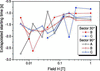Figure 7 Single-logarithmic plot of the extrapolated virtual starting times. The starting times are negative since on the time axis they are positioned before the trigger point, which by definition has the time t = 0'.

The curves show that there is a general tendency of the virtual starting times to move closer to the trigger point (i.e. the point t = 0' where the field is actually zero), if the magnetic field during the field-on period was higher. At high fields, which are connected with a duration of the field-down ramp of the magnet of up to 3 s, the extrapolated times of 1 to 1.5 s are certainly too short. Obviously, in this case the diminishing magnetic field still continued to retain the ordering for a while, causing a delay of the actual decay. On the other hand, at low fields where the length of the field-down ramp was much shorter than 1 s, the large extrapolation results of 1.5 to 2.5 s suggest that this very first part of the decay process must take place according to a decay law much faster than exponential.

The decay curves also revealed a direct indication for a different, faster decay mechanism at this early time. When fitting equation (2), it was observed that at all fields the slope of the intensity curves was steeper than exponential during the first 200 to 400 ms after the trigger point (i.e. the first 2 to 4 points in Fig. 1), while for the rest of the curves the exponential approximation was nearly perfect. However, the available experimental data did not allow a precise determination of this faster decay function.

### 4. Relaxation mechanisms

In the concentrated Co-ferrofluid, field induced correlations between nanoparticles have been found to decay exponentially within a characteristic time of a few seconds. The observed relaxation times τ could be governed by the Brownian rotation of locally ordered domains of volume VD in a medium of viscosity η with a fixed dipole moment according to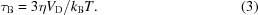kB is Boltzmann's constant and T is the temperature. For the present case of η = 0.2 Pa s, Brownian relaxation times between 1–5 s would imply aggregates of lateral sizes of the order of 100–150 nm which corresponds to the estimates (Wiedenmann et al., 2003) from the widths of the correlation peaks. The time constants fitted for the decay of the structure peaks were found to increase with increasing strength of the inducing magnetic field which then implies an increasing size of ordered domains. This must be related to dipolar interaction between individual particle moments m0 at a distance σ, the energy EDD of which is given by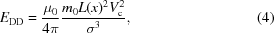and is expected to increase with increasing alignment of the moments along an external magnetic field as determined by the Langevin function L(x). Vc is the core volume of the particles and µ0 is the permeability constant.

As a second relaxation mechanism, the free rotation of the magnetic dipole moment inside a fixed particle has to be considered. For this Néel rotation', the characteristic time τN is given by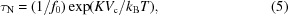where K is the shape-anisotropy constant and f0 is of the order of 109 Hz. Using the structural parameters of the sample MFT (Wiedenmann & Heinemann, 2005) K = 2.6 × 105 J m−3 for f.c.c. Co and core radius Rc = 4.4 ± 0.2 nm, Néel relaxation times are expected in the range between 0.2 and 80 s, which is of the same order of magnitude. Brownian rotation of ordered domains and Néel rotation of single moments can occur simultaneously, which should then lead to an apparent characteristic time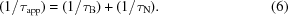While the main part of the decay is characterized by a single exponential course, in the very beginning the decay appears to take place faster, indicating that a different decay mechanism should be active. This is a strong indication that not all of the particle moments are involved in the locally ordered domains. For such individual core-shell particles, the Brownian rotation would be by four orders of magnitude faster than observed here. In fact, recent dynamic stroboscopic SANS studies gave a clear confirmation of this fast mechanism (Wiedenmann et al., 2006a).

### 5. Conclusion

We have shown that the stroboscopic time-resolved SANS technique is a powerful tool for investigating reversible periodical processes. The relaxation times fitted for the decay of the structure peaks were found to increase with increasing strength of the inducing magnetic field, showing the influence of dipolar interactions on the structure formation. The mechanism is governed by the rotation of domains formed by hexagonal ordering of core-shell particles and subsequent transformation to uncorrelated segments of chains. The time behaviour at the very beginning gives some hint of a second, much faster process of relaxation.

### ReferencesJOURNAL OFAPPLIEDCRYSTALLOGRAPHY